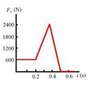# Velocity from a force time graph

• TheFluffyDuck
In summary, the problem asks for the speed at which a woman leaves the ground during a vertical jump, taking into account the force of the floor and other forces acting on her. The solution involves calculating the impulse from the area under the graph, considering the woman's mass and weight, and finding the net force acting on her.

## Homework Statement

The figure is a graph of the force exerted by the floor on a woman making a vertical jump.
At what speed does she leave the ground? Hint: The force of the floor is not the only force acting on the woman.

## The Attempt at a Solution

I am completely lost with this question. First I derived her mass from the 600N from the graph. Which was about 61.22kg

Then I calculated the Impusle from the area underthe graph, but where from? All the area or minus the forces of gravity? then I used:

Impulse= m(delta v)

But I am not getting anywhere :(

#### Attachments

•image022.jpg.jpeg
4 KB · Views: 1,345
Starting with this: Impulse= m(delta v)

Then delta v = Impulse/m, and delta v = v - vo, and vo = ?

Im guessing Vo is zero becasue she is jumping straight up?

Do I calculate Impulse to be the entire area under the graph or just a certain segment under the graph?

TheFluffyDuck said:
Im guessing Vo is zero becasue she is jumping straight up?
Correct. Standing she starts are rest.

Do I calculate Impulse to be the entire area under the graph or just a certain segment under the graph?
Weight which is a force, does not provide for acceleration. One has to consider the force in excess of the weight.

Astronuc said:
Correct. Standing she starts are rest.

Weight which is a force, does not provide for acceleration. One has to consider the force in excess of the weight.